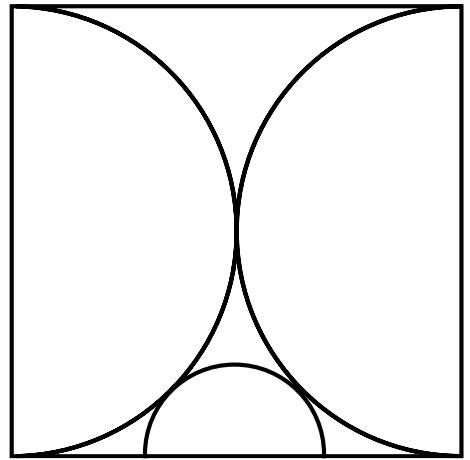# Free GRE Practice Questions

Question 1 of 1
ID: GRE-MCQ-20
Section: Quantitative Reasoning - Multiple Choice with one answer
Topics: Geometry; Circles
Difficulty level: Hard

(Practice Mode: Single selected Question » Back to Overview)In the square shown above, the side is 4 units. The three semicircles with their diameters along the sides of the square touch each other as shown. What is the diameter of the smaller semicircle?

A$\sqrt{2}-1$
B$2\sqrt{2}-2$
C$\sqrt{2}$
D$4\sqrt{2}-4$
E$\sqrt{3}$
•